# js实现波浪水波纹效果原理附源码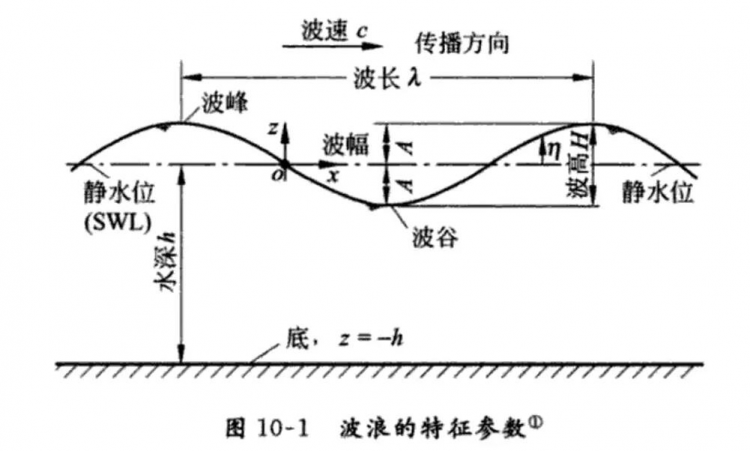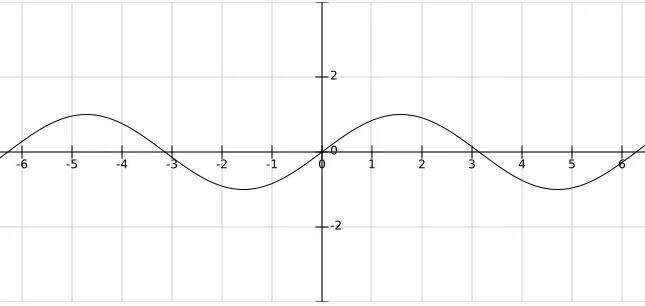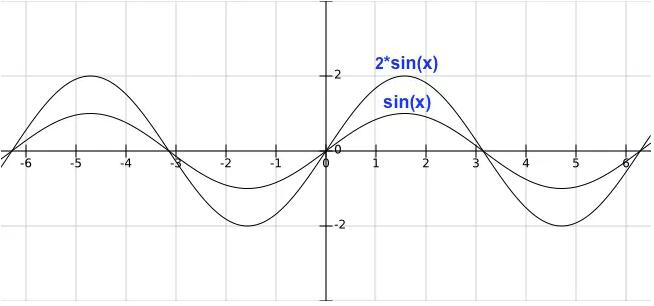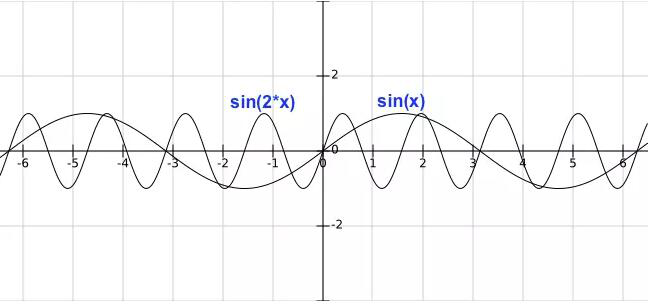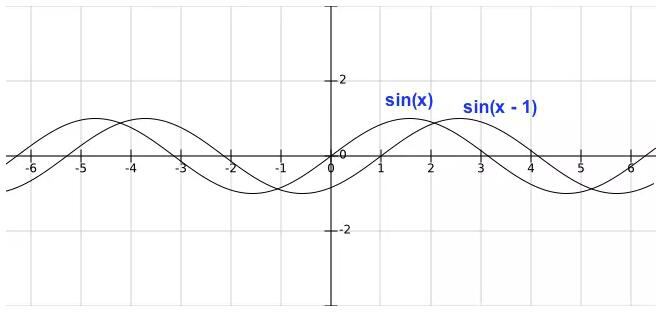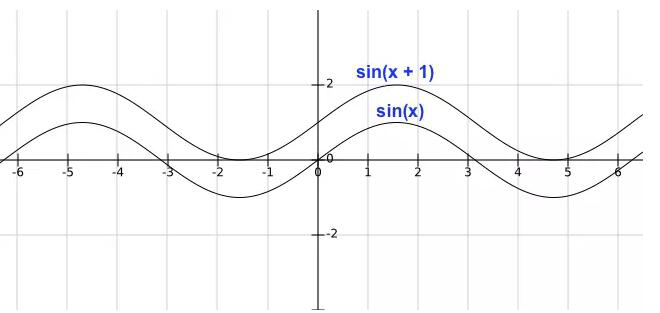振幅：控制波浪的高度
周期：控制波浪的宽度
相移：控制波浪的水平移动
垂直位移：控制水位的高度

componentDidMount() {
const self = this;
const canvas = this.refs.canvas;
canvas.height = 500;
canvas.width = 500;
this.canvas = canvas;
this.canvasWidth = canvas.width;
this.canvasHeight = canvas.height;
const ctx = canvas.getContext('2d');
this.drawSin(ctx);
}
render() {
return (
<div className="content page">
<canvas ref="canvas"></canvas>
</div>
);
}

drawSin(ctx) {
const points = [];
const canvasWidth = this.canvasWidth;
const canvasHeight = this.canvasHeight;
const startX = 0;
const waveWidth = 0.05; // 波浪宽度,数越小越宽
const waveHeight = 20; // 波浪高度,数越大越高
const xOffset = 0; // 水平位移

ctx.beginPath();
for (let x = startX; x < startX + canvasWidth; x += 20 / canvasWidth) {
const y = waveHeight * Math.sin((startX + x) * waveWidth + xOffset);
points.push([x, (canvasHeight / 2) + y]);
ctx.lineTo(x, (canvasHeight / 2) + y);
}
ctx.lineTo(canvasWidth, canvasHeight);
ctx.lineTo(startX, canvasHeight);
ctx.lineTo(points, points);
ctx.stroke();
}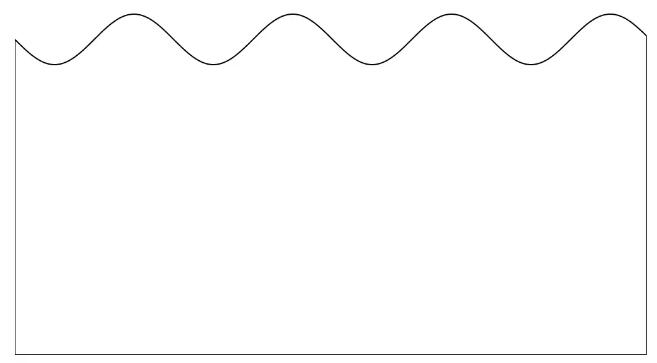componentDidMount() {
...
this.xOffset = 0;  // 初始偏移
this.speed = 0.1;  // 偏移速度
requestAnimationFrame(this.draw.bind(this, canvas));
}

draw() {
const canvas = this.canvas;
const ctx = canvas.getContext('2d');
ctx.clearRect(0, 0, canvas.width, canvas.height);
// 曲线绘制
this.drawSin(ctx, this.xOffset);
this.xOffset += this.speed;
requestAnimationFrame(this.draw.bind(this));
}

drawSin(ctx, xOffset = 0) {
...
}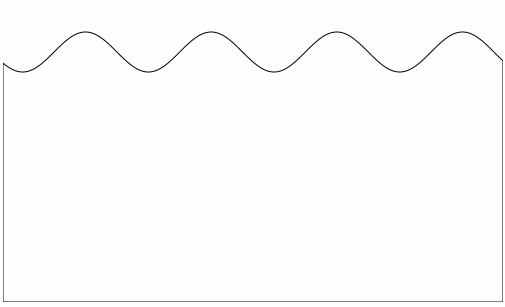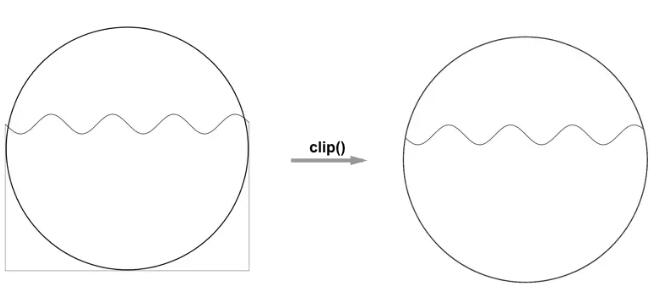draw() {
...
if (!this.isDrawCircle) {
this.drawCircle(ctx);
}
this.drawSin(ctx, this.xOffset);
this.xOffset += this.speed;
requestAnimationFrame(this.draw.bind(this));
}

drawCircle(ctx) {
const r = this.canvasWidth / 2;
const lineWidth = 5;
const cR = r - (lineWidth);
ctx.lineWidth = lineWidth;
ctx.beginPath();
ctx.arc(r, r, cR, 0, 2 * Math.PI);
ctx.stroke();
ctx.clip();
this.isDrawCircle = true;
}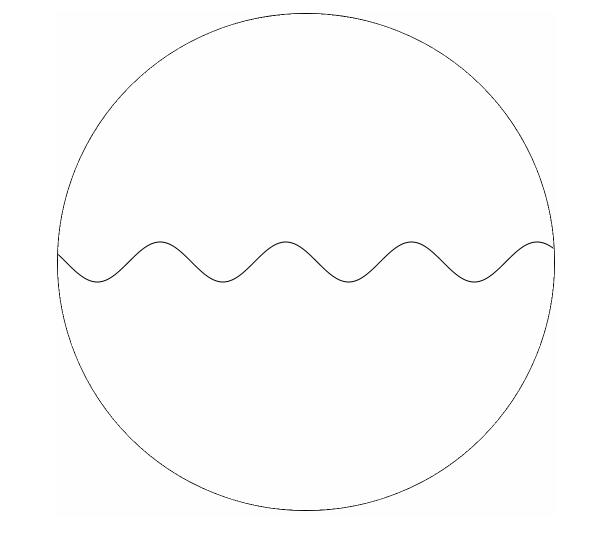componentDidMount() {
...
this.xOffset = 0;
this.speed = 0.1;
// 水位数值
this.rangeValue = 0.6;
// 初始水位
this.nowRange = 0;
requestAnimationFrame(this.draw.bind(this, canvas));
}

draw() {
...
this.drawSin(ctx, this.xOffset, this.nowRange);
this.xOffset += this.speed;
if (this.nowRange < this.rangeValue) {
this.nowRange += 0.01;
}
requestAnimationFrame(this.draw.bind(this));
}

drawCircle(ctx) {
...
}

drawSin(ctx, xOffset = 0, nowRange = 0) {
...
for (let x = startX; x < startX + canvasWidth; x += 20 / canvasWidth) {
const y = waveHeight * Math.sin((startX + x) * waveWidth + xOffset);
points.push([x, (1 - nowRange) * canvasHeight + y]);
ctx.lineTo(x, (1 - nowRange) * canvasHeight + y);
}
...
}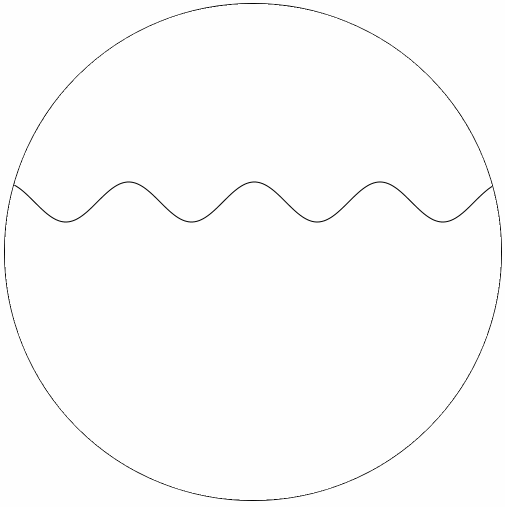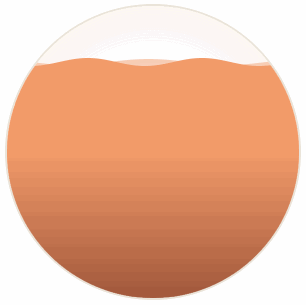jquery视频教程从入门到精通，课程主要包含：jquery选择器、jquery事件、jquery文档操作、动画、Ajax、jquery插件的制作、jquery下拉无限加载插件的制作等等......
VUE视频教程是目前最火的前端框架之一，就业薪资很高，本课程教您如何快速学会VUE并应用到实战，教你如何解决内存泄漏，常用UI库的使用，自己封装组件，正式上线白屏问题，性能优化等。对正在工作当中或打算学习VUE高薪就...
React是目前最火的前端框架，就业薪资很高，本课程教您如何快速学会React并应用到实战，教你如何解决内存泄漏，常用UI库的使用，自己封装组件，正式上线白屏问题，性能优化等。对正在工作当中或打算学习React高薪就...

React是前端最火的框架之一，就业薪资很高，本课程教您如何快速学会React并应用到实战，对正在工作当中或打算学习React高薪就业的你来说，那么这门课程便是你手中的葵花宝典。
HTML5基础入门视频教程，教学思路清晰，简单易学必会。适合人群：创业者，只要会打字，对互联网编程感兴趣都可以学。课程概述：该课程主要讲解HTML(学习HTML5的必备基础语言)、CSS3、Javascript(学习...
React和VUE是目前最火的前端框架，就业薪资很高，本课程教您如何快速学会React和VUE并应用到实战，教你如何解决内存泄漏，常用库的使用，自己封装组件，正式上线白屏问题，性能优化等。对正在工作当中或打算学习Re...